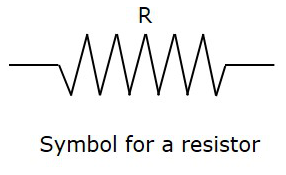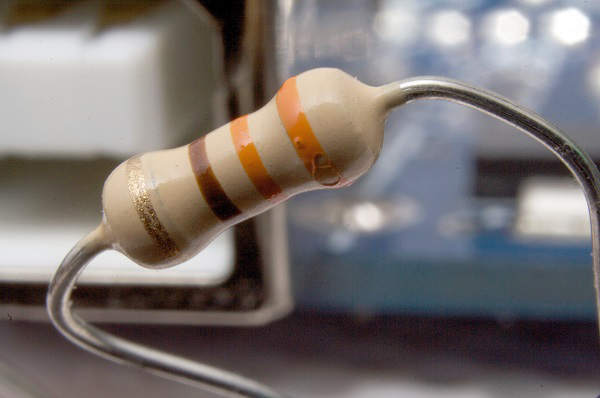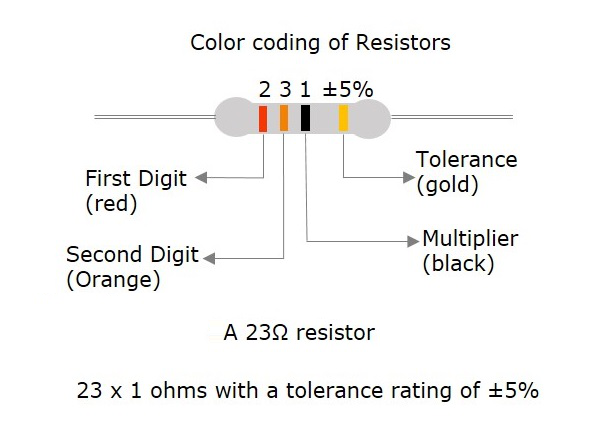# Basic Electronics - Resistors

Resist is the word which means “to oppose”. Resistance is the property of opposing the flow of electrons, in a conductor or a semiconductor. A Resistor is an electronic component which has the property of resistance.

## Symbol and Units

The symbol for a Resistor is as shown below.The units of resistance is Ohms, which is indicated by Ω (omega).

The formula for resistance is

R = V/I

Where V is Voltage and I is Current. It would really be difficult to manufacture the resistors with each and every value. Hence, few values are chosen and the resistors of such values are only manufactured. These are called as “Preferred Values”. In practice, the resistors with near values are chosen to match the required applications. This is how a practical resistor looks like −## Color Coding

A process called color coding is used to determine the value of resistance for a resistor, just as shown in the above figure. A resistor is coated with four color bands where each color determines a particular value. The below table shows a list of values which each color indicates.

COLOUR DIGIT MULTIPLIER TOLERANCE
Black 0 100 = 1
Brown 1 101 = 10 1
Red 2 102 = 100 2
Orange 3 103 = 1000
Yellow 4 104 = 10000
Green 5 105 = 100000 0.5
Blue 6 106 = 1000000 0.25
Violet 7 107 = 10000000 0.1
Gray 8 108 = 100000000
White 9 109 = 1000000000
Gold 10-1 = 0.1 5
Silver 10-2 = 0.01 10
(none) 20

The first two colored bands indicate the first and second digit of the value and the third color band represents the multiplier (number of zeroes added). The fourth color band indicates the tolerance value.

Tolerance is the range of value up to which a resistor can withstand without getting destroyed. This is an important factor. The following figure shows how the value of a resistor is determined by color code.The five color band resistors are manufactured with tolerance of 2% and 1% and also for other high accuracy resistors. In these five band resistors, the first three bands represent digits, fourth one indicates multiplier and the fifth represents tolerance.

Let us look at an example to understand the color coding process.

Example 1 − Determine the value of a resistor with a color code yellow, blue, orange and silver.

Solution − The value of yellow is 4, blue is 6, orange is 3 which represents multiplier. Silver is ±10 which is the tolerance value.

Hence the value of the resistor is 46×103 = 46kΩ

The maximum resistance value for this resistor is

46kΩ or 46000Ω + 10% = 46000 + 4600 = 50600Ω = 50.6kΩ

The minimum resistance value for this resistor is

46kΩ or 46000Ω - 10% = 46000 - 4600 = 41400Ω = 41.4kΩ

After having gone through different details regarding resistors, we have some terms to learn. Also we have to deal with different behaviors of a resistor for few types of connections.

## Important Terms

There are a few terms which we need to discuss before going into the type of resistors we have. One needs to get introduced to these terms at this stage and can understand them as we progress further.

### Resistance

Resistance is the property of a resistor that opposes the flow of current. When alternating current goes through a resistance, a voltage drop is produced that is in-phase with the current.

• Indication − R

• Units − Ohms

• Symbol − Ω

Along with resistance, there are other important terms, called as reactance and impedance.

### Reactance

The resistance offered to the alternating current because of the capacitances and inductances present in the circuit, can be understood as reactance. When alternating current goes through a pure reactance, a voltage drop is produced that is 90°out of phase with the current.

Depending upon the phase i.e., +90° or -90° the reactance can be termed as inductive reactance or capacitive reactance.

• Indication − X

• Units − Ohms

• Symbol − Ω

### Impedance

Impedance is the effective resistance to alternating current arising from the combined effects of ohmic resistance and reactance. When alternating current goes through an impedance, a voltage drop is produced which is somewhere between 0°to 90°out of phase with the current.

• Indication − I

• Units − Ohms

• Symbol − Ω

### Conductance

This is the ability of a material to conduct electricity. It is the reciprocal of resistance.

• Indication − G

• Units − Mhos

• Symbol −